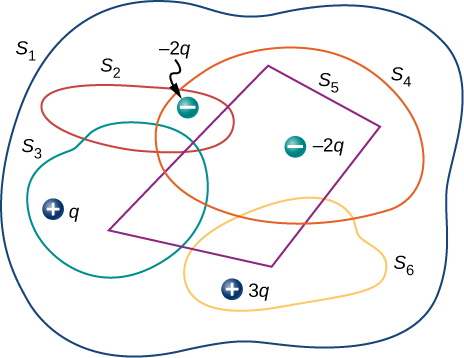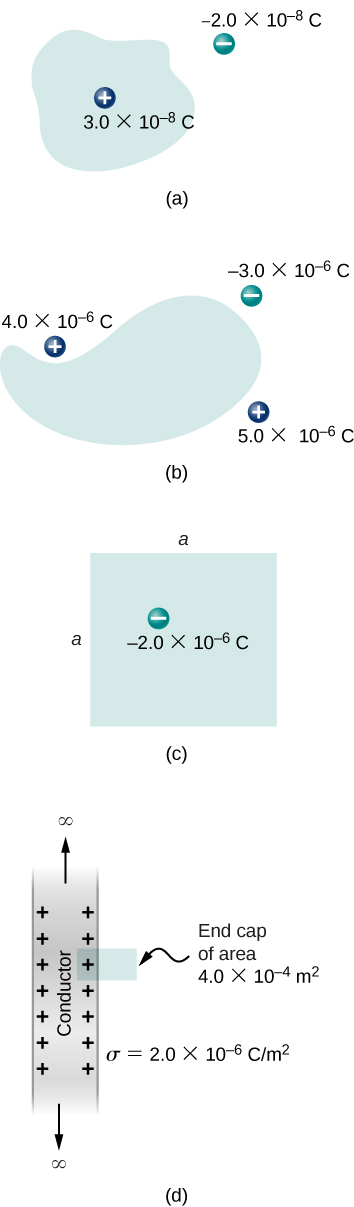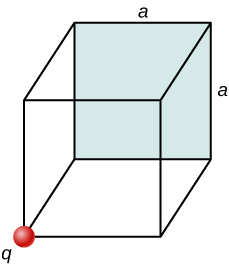# 6.2 Explaining gauss’s law  (Page 3/4)

 Page 3 / 4

## Gauss’s law

The flux $\text{Φ}$ of the electric field $\stackrel{\to }{\text{E}}$ through any closed surface S (a Gaussian surface) is equal to the net charge enclosed $\left({q}_{\text{enc}}\right)$ divided by the permittivity of free space $\left({\epsilon }_{0}\right):$

$\text{Φ}={\oint }_{S}\stackrel{\to }{\text{E}}·\stackrel{^}{\text{n}}\phantom{\rule{0.2em}{0ex}}dA=\frac{{q}_{\text{enc}}}{{\epsilon }_{0}}.$

To use Gauss’s law effectively, you must have a clear understanding of what each term in the equation represents. The field $\stackrel{\to }{\text{E}}$ is the total electric field at every point on the Gaussian surface. This total field includes contributions from charges both inside and outside the Gaussian surface. However, ${q}_{\text{enc}}$ is just the charge inside the Gaussian surface. Finally, the Gaussian surface is any closed surface in space. That surface can coincide with the actual surface of a conductor, or it can be an imaginary geometric surface. The only requirement imposed on a Gaussian surface is that it be closed ( [link] ).A Klein bottle partially filled with a liquid. Could the Klein bottle be used as a Gaussian surface?

## Electric flux through gaussian surfaces

Calculate the electric flux through each Gaussian surface shown in [link] .

## Strategy

From Gauss’s law, the flux through each surface is given by ${q}_{\text{enc}}\text{/}{\epsilon }_{0},$ where ${q}_{\text{enc}}$ is the charge enclosed by that surface.

## Solution

For the surfaces and charges shown, we find

1. $\text{Φ}=\frac{2.0\phantom{\rule{0.2em}{0ex}}\mu \text{C}}{{\epsilon }_{0}}=2.3\phantom{\rule{0.2em}{0ex}}×\phantom{\rule{0.2em}{0ex}}{10}^{5}\phantom{\rule{0.2em}{0ex}}\text{N}·{\text{m}}^{2}\text{/C}\text{.}$
2. $\text{Φ}=\frac{-2.0\phantom{\rule{0.2em}{0ex}}\mu \text{C}}{{\epsilon }_{0}}=-2.3\phantom{\rule{0.2em}{0ex}}×\phantom{\rule{0.2em}{0ex}}{10}^{5}\phantom{\rule{0.2em}{0ex}}\text{N}·{\text{m}}^{2}\text{/C}\text{.}$
3. $\text{Φ}=\frac{2.0\phantom{\rule{0.2em}{0ex}}\mu \text{C}}{{\epsilon }_{0}}=2.3\phantom{\rule{0.2em}{0ex}}×\phantom{\rule{0.2em}{0ex}}{10}^{5}\phantom{\rule{0.2em}{0ex}}\text{N}·{\text{m}}^{2}\text{/C}\text{.}$
4. $\text{Φ}=\frac{-4.0\phantom{\rule{0.2em}{0ex}}\mu \text{C}+6.0\phantom{\rule{0.2em}{0ex}}\mu \text{C}-1.0\phantom{\rule{0.2em}{0ex}}\mu \text{C}}{{\epsilon }_{0}}=1.1\phantom{\rule{0.2em}{0ex}}×\phantom{\rule{0.2em}{0ex}}{10}^{5}\phantom{\rule{0.2em}{0ex}}\text{N}·{\text{m}}^{2}\text{/C}\text{.}$
5. $\text{Φ}=\frac{4.0\phantom{\rule{0.2em}{0ex}}\mu \text{C}+6.0\phantom{\rule{0.2em}{0ex}}\mu \text{C}-10.0\phantom{\rule{0.2em}{0ex}}\mu \text{C}}{{\epsilon }_{0}}=0.$

## Significance

In the special case of a closed surface, the flux calculations become a sum of charges. In the next section, this will allow us to work with more complex systems.

Check Your Understanding Calculate the electric flux through the closed cubical surface for each charge distribution shown in [link] .

a. $3.4\phantom{\rule{0.2em}{0ex}}×\phantom{\rule{0.2em}{0ex}}{10}^{5}\phantom{\rule{0.2em}{0ex}}\text{N}·{\text{m}}^{2}\text{/C;}$ b. $-3.4\phantom{\rule{0.2em}{0ex}}×\phantom{\rule{0.2em}{0ex}}{10}^{5}\phantom{\rule{0.2em}{0ex}}\text{N}·{\text{m}}^{2}\text{/C;}$ c. $3.4\phantom{\rule{0.2em}{0ex}}×\phantom{\rule{0.2em}{0ex}}{10}^{5}\phantom{\rule{0.2em}{0ex}}\text{N}·{\text{m}}^{2}\text{/C;}$ d. 0

Use this simulation to adjust the magnitude of the charge and the radius of the Gaussian surface around it. See how this affects the total flux and the magnitude of the electric field at the Gaussian surface.

## Summary

• Gauss’s law relates the electric flux through a closed surface to the net charge within that surface,
$\text{Φ}={\oint }_{S}\stackrel{\to }{\text{E}}·\stackrel{^}{\text{n}}\phantom{\rule{0.2em}{0ex}}dA=\frac{{q}_{\text{enc}}}{{\epsilon }_{0}},$

where ${q}_{\text{enc}}$ is the total charge inside the Gaussian surface S .
• All surfaces that include the same amount of charge have the same number of field lines crossing it, regardless of the shape or size of the surface, as long as the surfaces enclose the same amount of charge.

## Conceptual questions

Two concentric spherical surfaces enclose a point charge q . The radius of the outer sphere is twice that of the inner one. Compare the electric fluxes crossing the two surfaces.

Since the electric field vector has a $\frac{1}{{r}^{2}}$ dependence, the fluxes are the same since $A=4\pi {r}^{2}$ .

Compare the electric flux through the surface of a cube of side length a that has a charge q at its center to the flux through a spherical surface of radius a with a charge q at its center.

(a) If the electric flux through a closed surface is zero, is the electric field necessarily zero at all points on the surface? (b) What is the net charge inside the surface?

a. no; b. zero

Discuss how Gauss’s law would be affected if the electric field of a point charge did not vary as $1\text{/}{r}^{2}.$

Discuss the similarities and differences between the gravitational field of a point mass m and the electric field of a point charge q .

Both fields vary as $\frac{1}{{r}^{2}}$ . Because the gravitational constant is so much smaller than $\frac{1}{4\pi {\epsilon }_{0}}$ , the gravitational field is orders of magnitude weaker than the electric field.

Discuss whether Gauss’s law can be applied to other forces, and if so, which ones.

Is the term $\stackrel{\to }{E}$ in Gauss’s law the electric field produced by just the charge inside the Gaussian surface?

No, it is produced by all charges both inside and outside the Gaussian surface.

Reformulate Gauss’s law by choosing the unit normal of the Gaussian surface to be the one directed inward.

## Problems

Determine the electric flux through each surface whose cross-section is shown below.Find the electric flux through the closed surface whose cross-sections are shown below.a. $\text{Φ}=3.39\phantom{\rule{0.2em}{0ex}}×\phantom{\rule{0.2em}{0ex}}{10}^{3}\phantom{\rule{0.2em}{0ex}}\text{N}·{\text{m}}^{2}\text{/}\text{C}$ ; b. $\text{Φ}=0$ ;
c. $\text{Φ}=-2.25\phantom{\rule{0.2em}{0ex}}×\phantom{\rule{0.2em}{0ex}}{10}^{5}\phantom{\rule{0.2em}{0ex}}\text{N}·{\text{m}}^{2}\text{/}\text{C}$ ;
d. $\text{Φ}=90.4\phantom{\rule{0.2em}{0ex}}\text{N}·{\text{m}}^{2}\text{/}\text{C}$

A point charge q is located at the center of a cube whose sides are of length a . If there are no other charges in this system, what is the electric flux through one face of the cube?

A point charge of $10\phantom{\rule{0.2em}{0ex}}\mu \text{C}$ is at an unspecified location inside a cube of side 2 cm. Find the net electric flux though the surfaces of the cube.

$\text{Φ}=1.13\phantom{\rule{0.2em}{0ex}}×\phantom{\rule{0.2em}{0ex}}{10}^{6}\phantom{\rule{0.2em}{0ex}}\text{N}·{\text{m}}^{2}\text{/C}$

A net flux of $1.0\phantom{\rule{0.2em}{0ex}}×\phantom{\rule{0.2em}{0ex}}{10}^{4}\phantom{\rule{0.2em}{0ex}}\text{N}·{\text{m}}^{2}\text{/C}$ passes inward through the surface of a sphere of radius 5 cm. (a) How much charge is inside the sphere? (b) How precisely can we determine the location of the charge from this information?

A charge q is placed at one of the corners of a cube of side a , as shown below. Find the magnitude of the electric flux through the shaded face due to q . Assume $q>0$ .Make a cube with q at the center, using the cube of side a . This would take four cubes of side a to make one side of the large cube. The shaded side of the small cube would be 1/24th of the total area of the large cube; therefore, the flux through the shaded area would be
$\text{Φ}=\frac{1}{24}\phantom{\rule{0.2em}{0ex}}\frac{q}{{\epsilon }_{0}}$ .

The electric flux through a cubical box 8.0 cm on a side is $1.2\phantom{\rule{0.2em}{0ex}}×\phantom{\rule{0.2em}{0ex}}{10}^{3}\phantom{\rule{0.2em}{0ex}}\text{N}·{\text{m}}^{2}\text{/C}\text{.}$ What is the total charge enclosed by the box?

The electric flux through a spherical surface is $4.0\phantom{\rule{0.2em}{0ex}}×\phantom{\rule{0.2em}{0ex}}{10}^{4}\phantom{\rule{0.2em}{0ex}}\text{N}·{\text{m}}^{2}\text{/C}\text{.}$ What is the net charge enclosed by the surface?

$q=3.54\phantom{\rule{0.2em}{0ex}}×\phantom{\rule{0.2em}{0ex}}{10}^{-7}\phantom{\rule{0.2em}{0ex}}\text{C}$

A cube whose sides are of length d is placed in a uniform electric field of magnitude $E=4.0\phantom{\rule{0.2em}{0ex}}×\phantom{\rule{0.2em}{0ex}}{10}^{3}\phantom{\rule{0.2em}{0ex}}\text{N/C}$ so that the field is perpendicular to two opposite faces of the cube. What is the net flux through the cube?

Repeat the previous problem, assuming that the electric field is directed along a body diagonal of the cube.

zero, also because flux in equals flux out

A total charge $5.0\phantom{\rule{0.2em}{0ex}}×\phantom{\rule{0.2em}{0ex}}{10}^{-6}\phantom{\rule{0.2em}{0ex}}\text{C}$ is distributed uniformly throughout a cubical volume whose edges are 8.0 cm long. (a) What is the charge density in the cube? (b) What is the electric flux through a cube with 12.0-cm edges that is concentric with the charge distribution? (c) Do the same calculation for cubes whose edges are 10.0 cm long and 5.0 cm long. (d) What is the electric flux through a spherical surface of radius 3.0 cm that is also concentric with the charge distribution?

what are waves
In physics, mathematics, and related fields, a wave is a propagating dynamic disturbance (change from equilibrium) of one or more quantities
Discuss how would orient a planar surface of area A in a uniform electric field of magnitude E0 to obtain (a) the maximum flux and (b) the minimum flux through the area.
I'm just doing the first 3 with this message. but thankyou for the time your obviously intending to support us with. viva la accumulation
Marcel
Find the net capacitance of the combination of series and parallel capacitors shown belo
what is ohm?
calculate ideal gas pressure of 0.300mol,v=2L T=40°c
what is principle of superposition
what are questions that are likely to come out during exam
what is electricity
watt is electricity.
electricity ka full definition with formula
Jyoti
If a point charge is released from rest in a uniform electric field will it follow a field line? Will it do so if the electric field is not uniform?
Maxwell's stress tensor is
Yes
doris
neither vector nor scalar
Anil
if 6.0×10^13 electrons are placed on a metal sphere of charge 9.0micro Coulombs, what is the net charge on the sphere
18.51micro Coulombs
ASHOK
Is it possible to find the magnetic field of a circular loop at the centre by using ampere's law?
Is it possible to find the magnetic field of a circular loop at it's centre?
yes
Brother
The density of a gas of relative molecular mass 28 at a certain temperature is 0.90 K kgmcube.The root mean square speed of the gas molecules at that temperature is 602ms.Assuming that the rate of diffusion of a gas in inversely proportional to the square root of its density,calculate the density of
A hot liquid at 80degree Celsius is added to 600g of the same liquid originally at 10 degree Celsius. when the mixture reaches 30 degree Celsius, what will be the total mass of the liquid?
Under which topic
doris
what is electrostatics
Study of charges which are at rest
himanshu

#### Get Jobilize Job Search Mobile App in your pocket Now!By Yasser IbrahimBy Madison ChristianBy Michael SagBy OpenStaxBy Mariah HauptmanBy Danielle StephensBy P. Wynn NormanBy Frank LevyBy Robert MurphyBy Cath Yu